# 半导体测试基础 - AC 参数测试

AC 测试确保 DUT 的时特性序满足其规格需求。

## 基本 AC 参数

### 建立时间（Setup Time）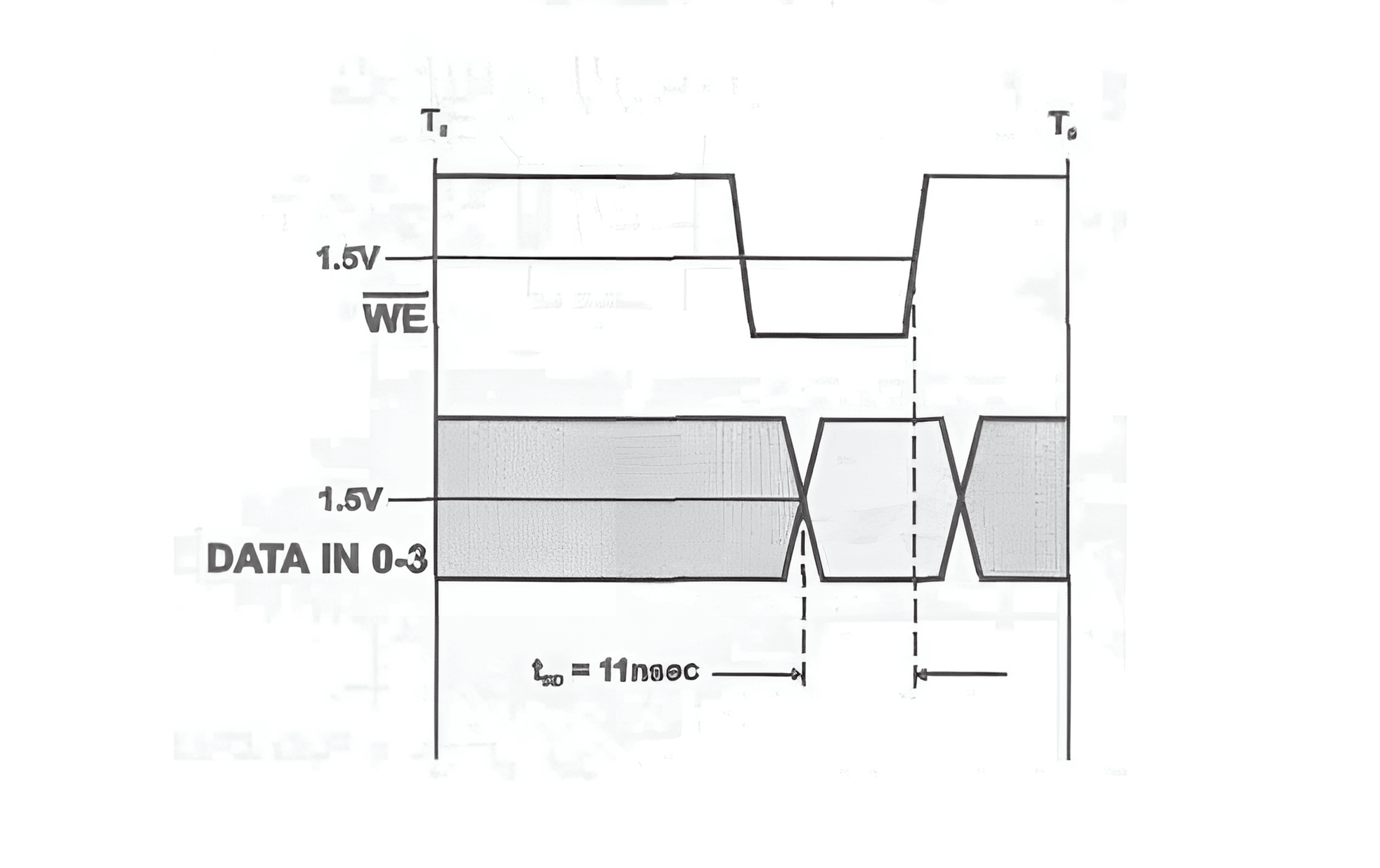Parameter Description Min Max Unit
$$t_{SD}$$ Data Set-Up to Write End 11 ns

### 保持时间（Hold Time）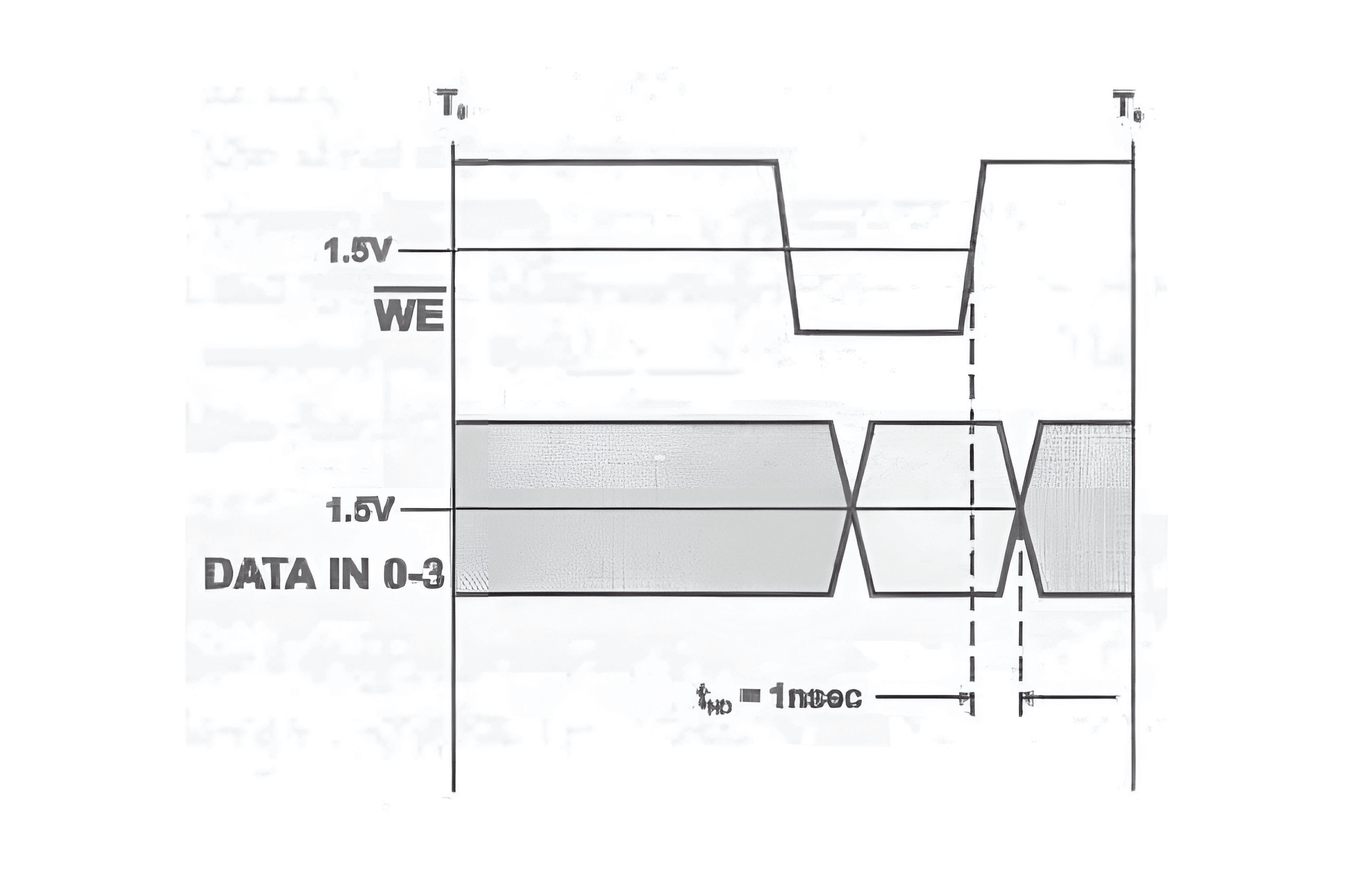Parameter Description Min Max Unit
$$t_{HD}$$ Data Hold from Write End 1 ns

### 传播时延（Propagation Delay）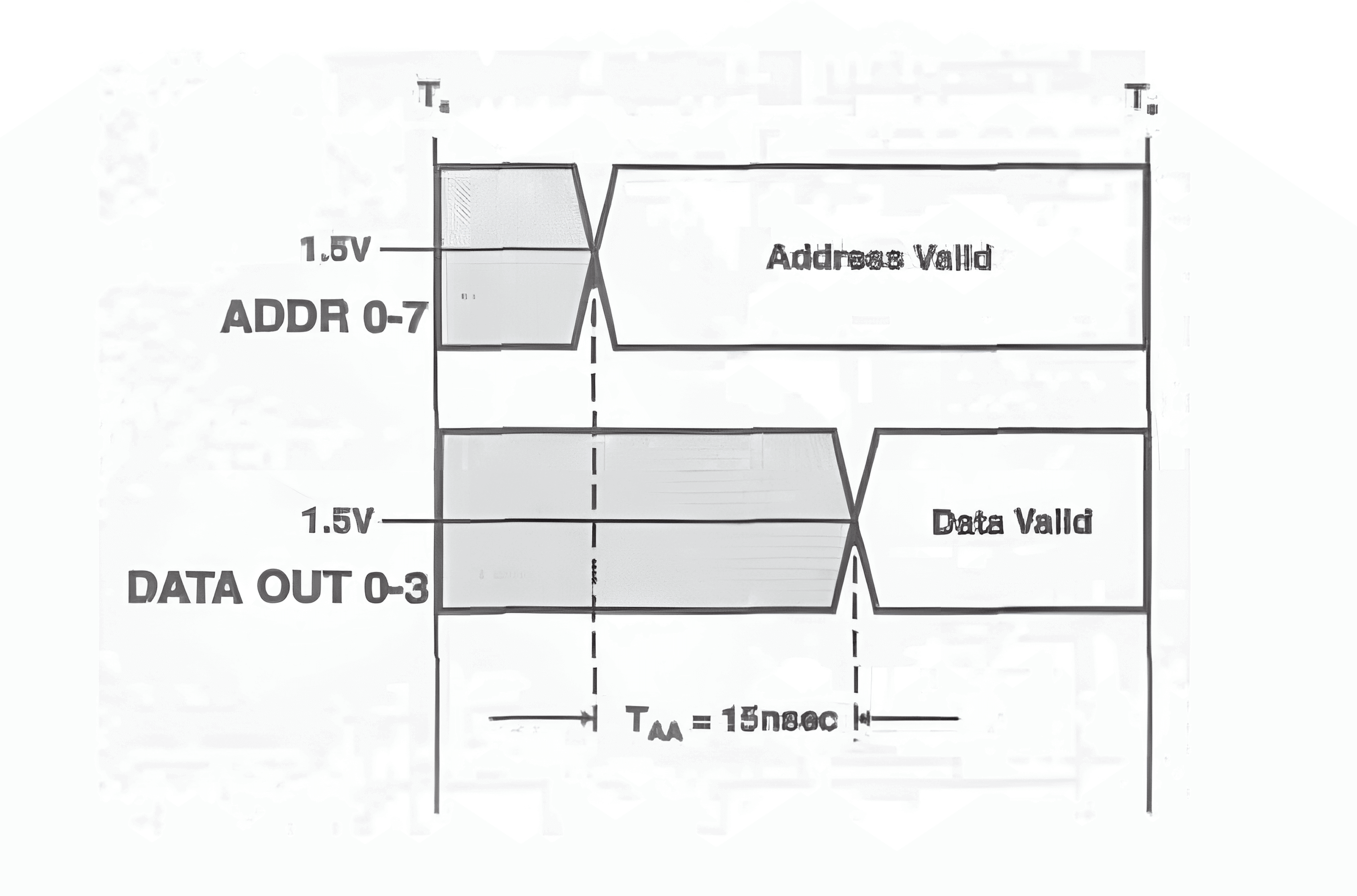Parameter Description Min Max Unit
$$t_{AA}$$ Address to Data Valid 15 ns

### 最小脉宽（Minimum Pulse Widths）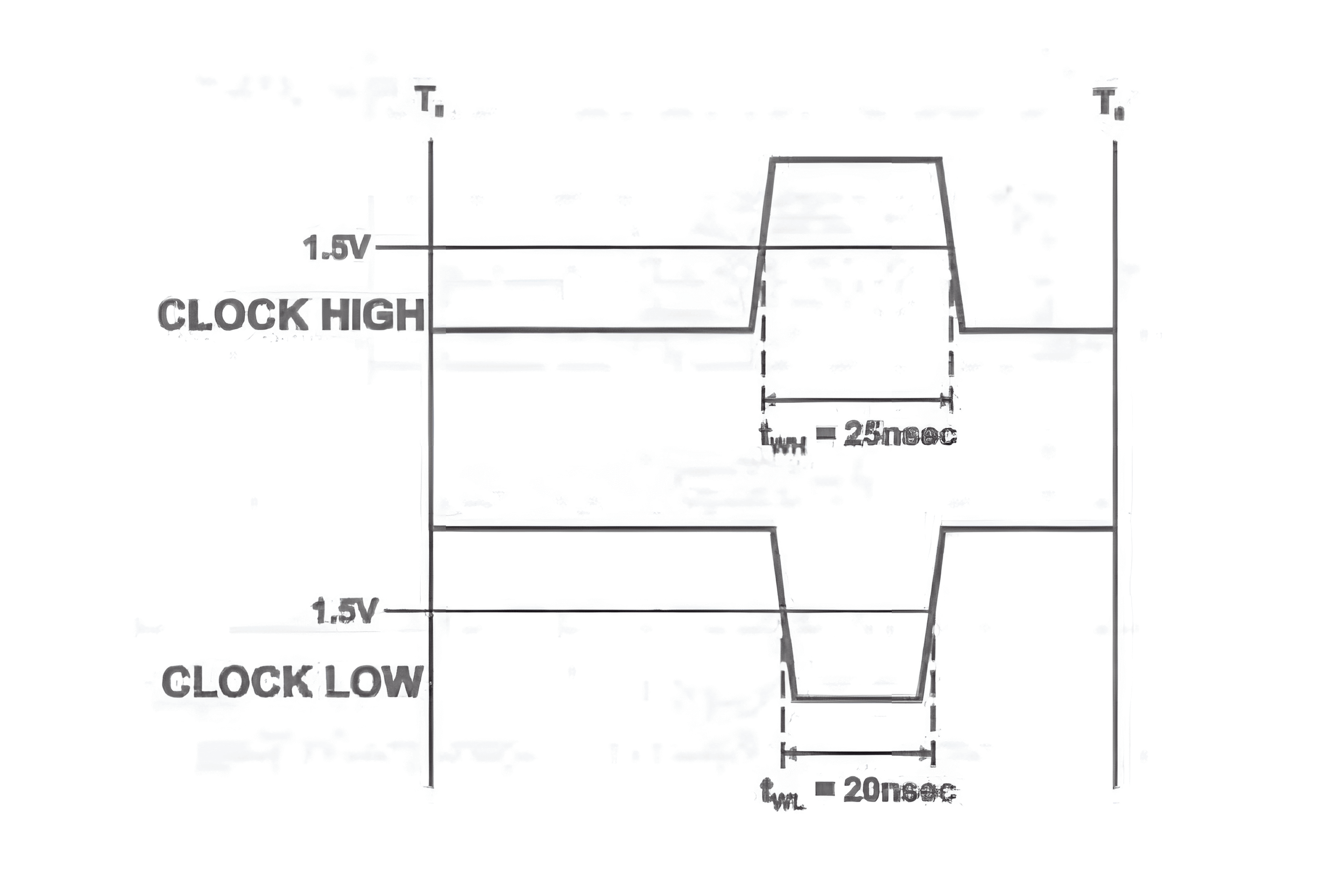Parameter Description Min Max Unit
$$t_{WL}$$ Minimum clock low time 20 ns
$$t_{WH}$$ Minimum clock high time 25 ns

### 最大频率（Maximum Frequency）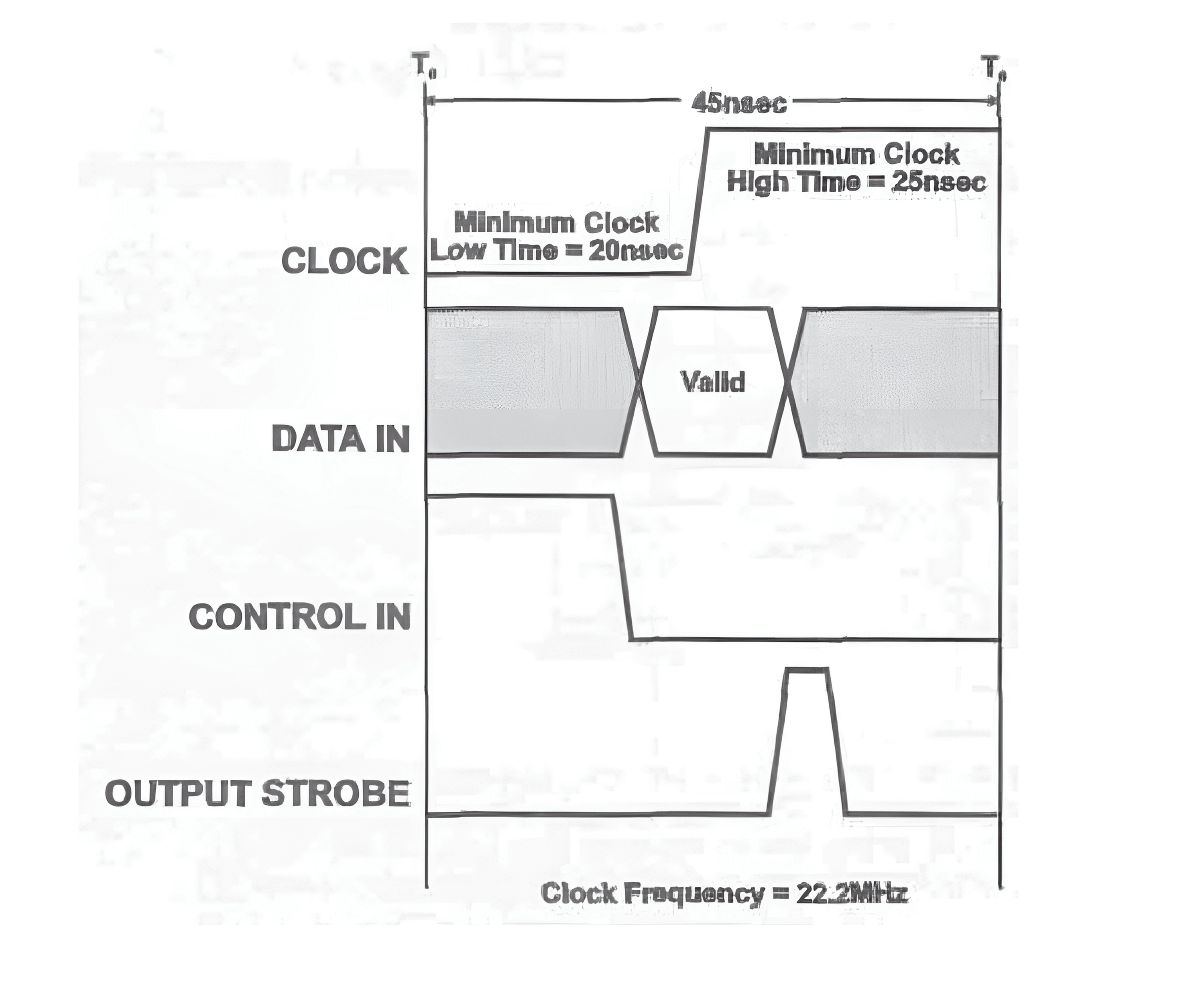Parameter Description Min Max Unit
$$f_{MAX}$$ Maximum clock frequency 22.2 MHz

### 输出使能时间（Output Enable Time）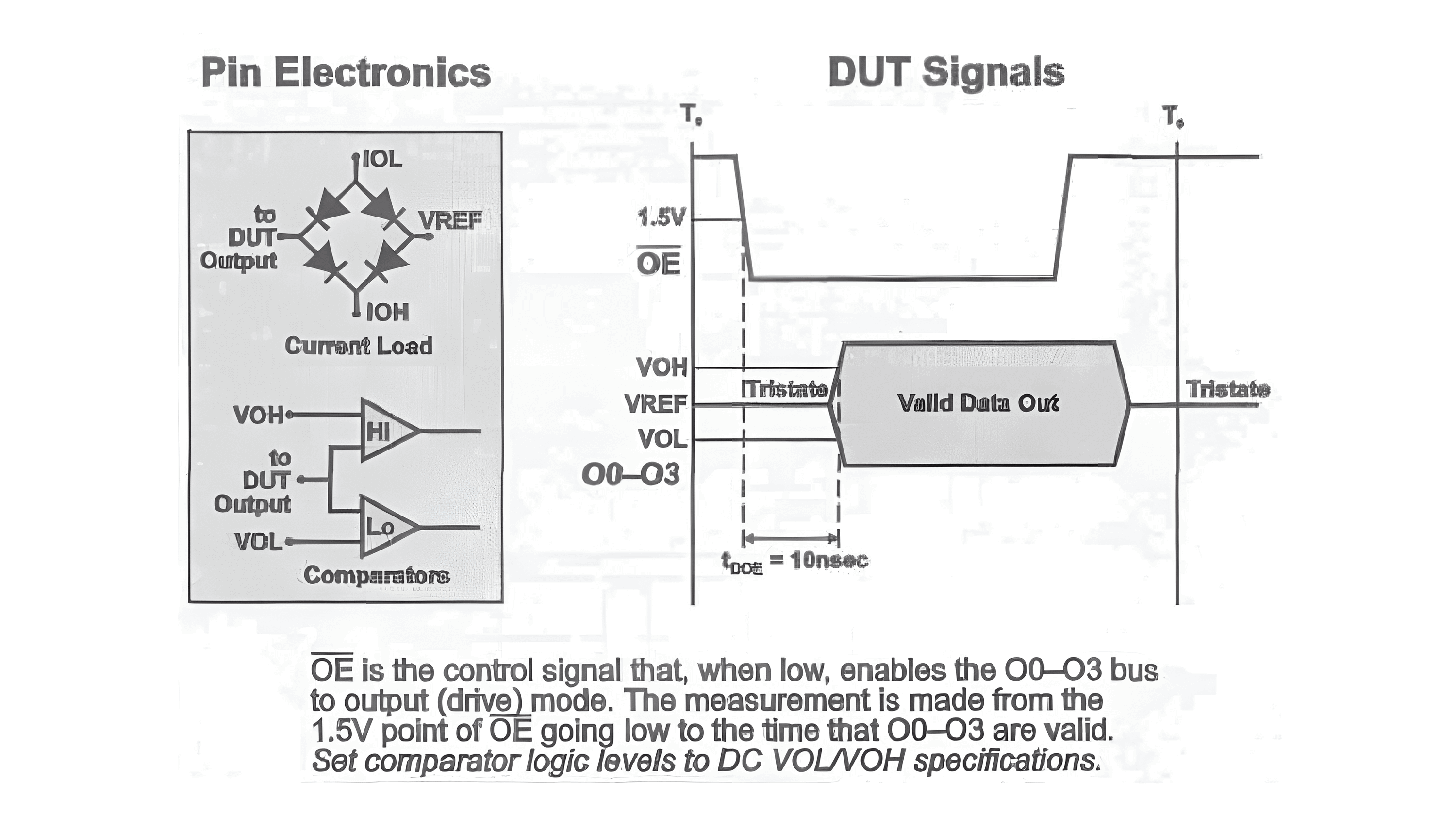Parameter Description Min Max Unit
$$t_{DOE}$$ OE LOW to Data Valid 10 ns

### 输出失能时间（Output Disable Time）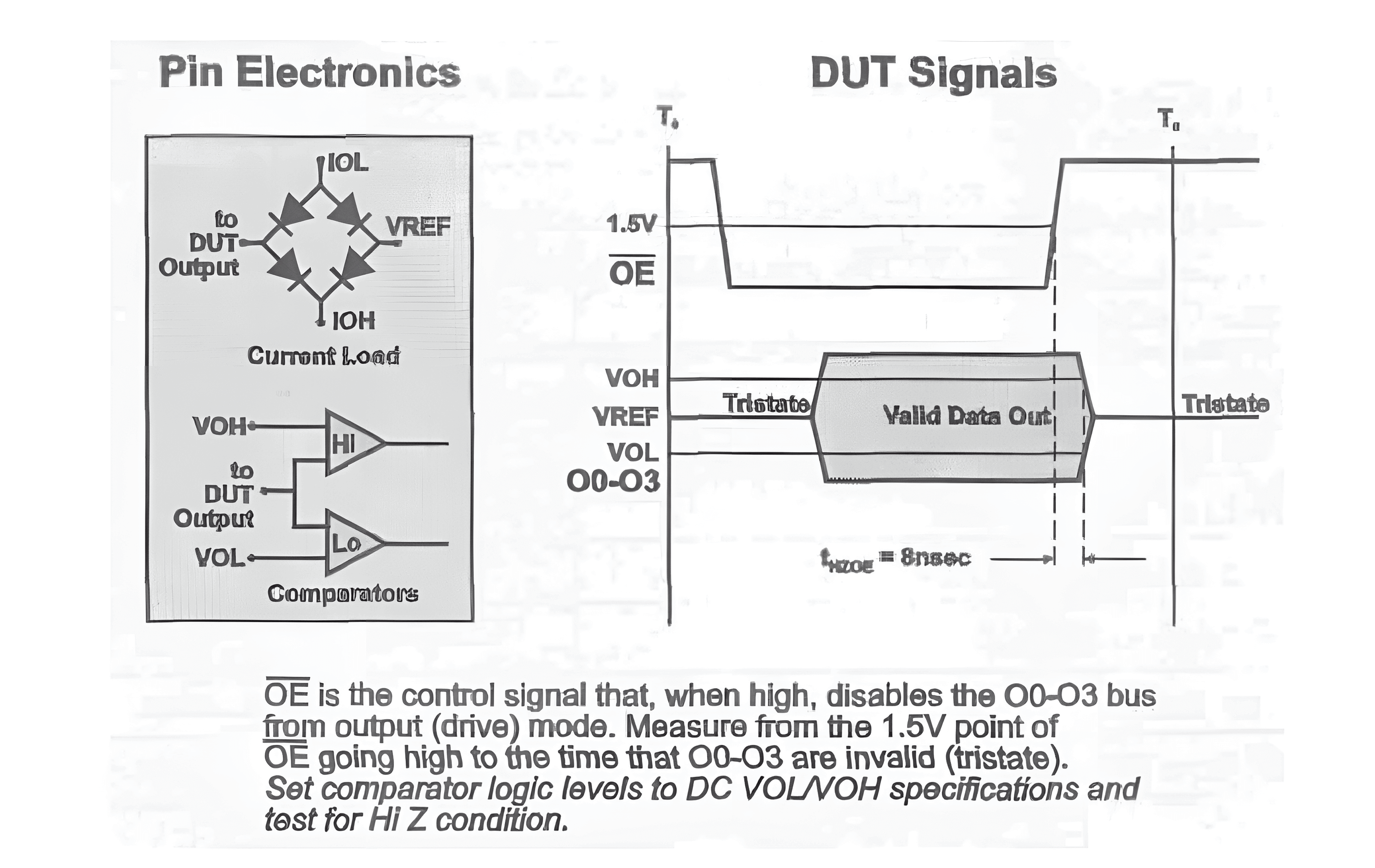Parameter Description Min Max Unit
$$t_{HZOE}$$ OE High to Data Valid 8 ns

## 时序参数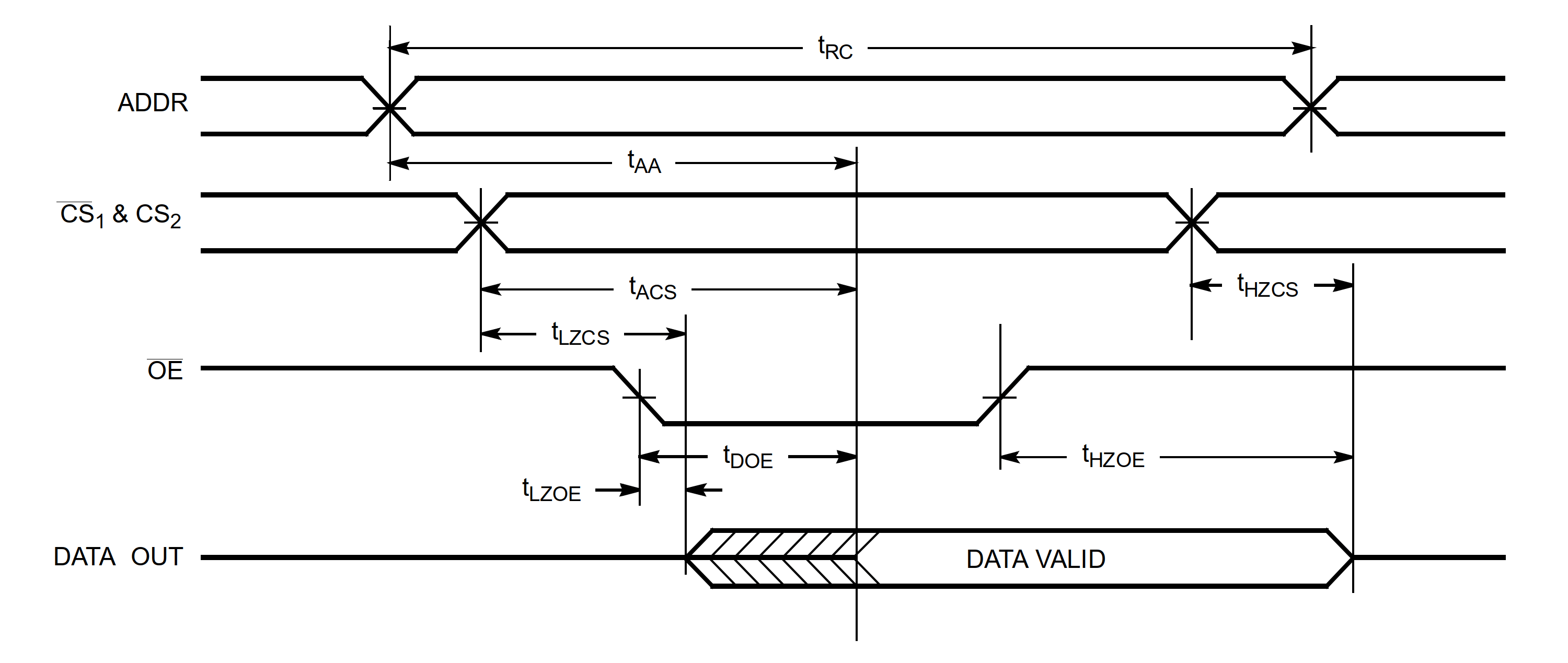Parameter Description Min Max Unit
$$t_{RC}$$ Read Cycle Time 15 ns
$$t_{AA}$$ Address to Data Valid 15 ns
$$t_{ACS}$$ Chip Select to Data Valid 10 ns
$$t_{DOE}$$ OE LOW to Data Valid 10 ns
$$t_{HZCS}$$ Chip Select to High Z 8 ns
$$t_{HZOE}$$ OE HIGH to High Z 8 ns
$$t_{LZCS}$$ Chip Select to Low Z 2 ns
$$t_{LZOE}$$ OE LOW to Low 2 ns
1. 首先由 $$t_{RC}$$ 参数确定写入周期的长度。
2. 确定哪个信号控制读取的功能。在上图的示例中，RAM 的数据输出是由 OE 的下降沿控制的。

### 写入周期时序（Write Cycle Timing）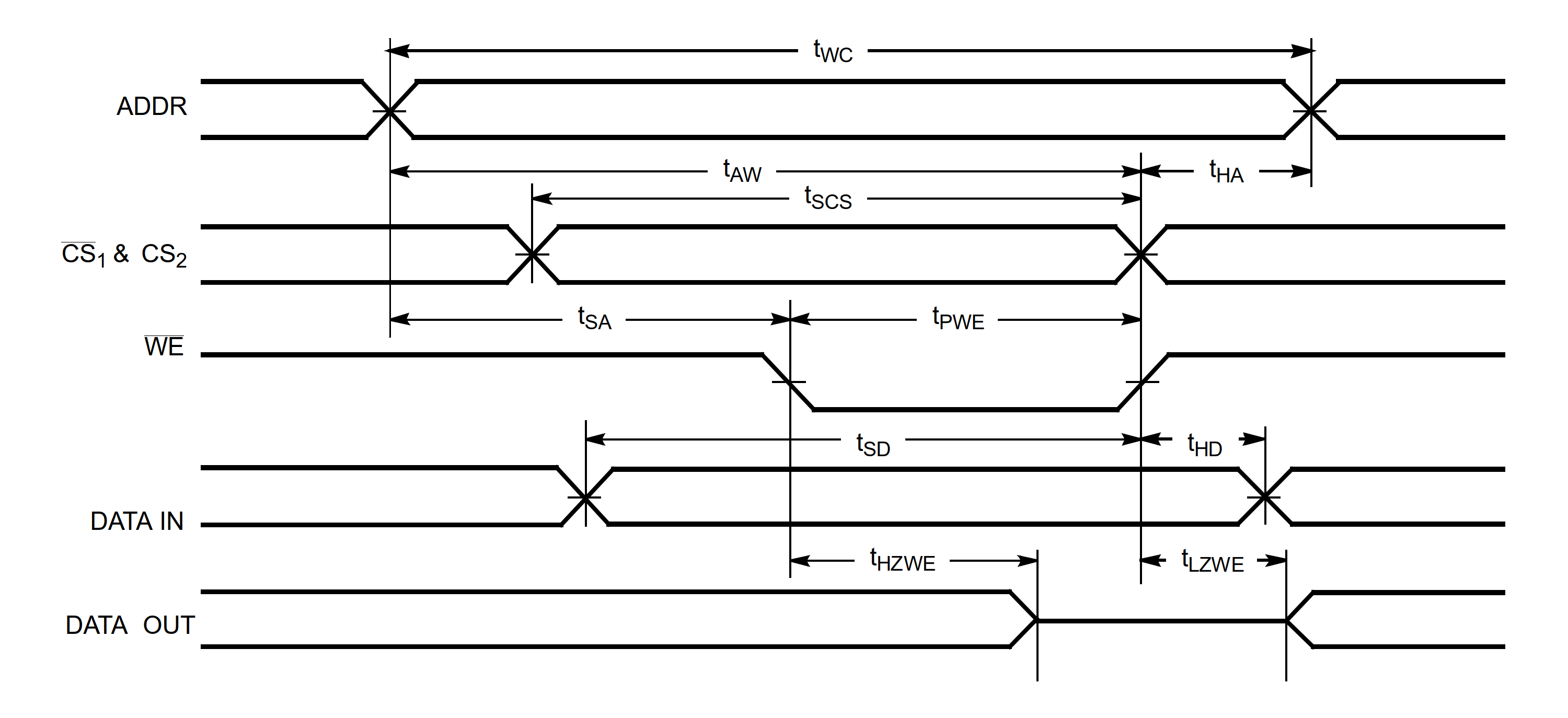Parameter Description Min Max Unit
$$t_{WC}$$ Write Cycle Time 15 ns
$$t_{HZWE}$$ WE LOW to High Z 8 ns
$$t_{LZWE}$$ WE HIGH to Low Z 2 ns
$$t_{PWE}$$ WE Pulse Width 11 ns
$$t_{SD}$$ Data Set-Up to Write End 11 ns
$$t_{HD}$$ Data Hold from Write End 1 ns
$$t_{SA}$$ Address Set-Up to Write Start 2 ns
$$t_{HA}$$ Address Hold from Write End 2 ns
$$t_{SCS}$$ CS LOW to Write End 11 ns
$$t_{AW}$$ Address Set-Up to Write End 13 ns
1. 首先由 $$t_{WC}$$ 参数确定写入周期的长度。
2. 确定哪个信号控制写入的功能。在上图的示例中，RAM 的数据读入是由 WE 的上升沿控制的。

## 参考与致谢

• 《The Fundamentals Of Digital Semiconductor Testing》